# The UNIVARIATE Procedure

### Computational Resources

Because the UNIVARIATE procedure computes quantile statistics, it requires additional memory to store a copy of the data in memory. By default, the MEANS, SUMMARY, and TABULATE procedures require less memory because they do not automatically compute quantiles. These procedures also provide an option to use a new fixed-memory quantiles estimation method that is usually less memory-intensive.

In the UNIVARIATE procedure, the only factor that limits the number of variables that you can analyze is the computer resources that are available. The amount of temporary storage and CPU time required depends on the statements and the options that you specify. To calculate the computer resources the procedure needs, let

 N be the number of observations in the data set V be the number of variables in the VAR statement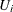be the number of unique values for the ith variable

Then the minimum memory requirement in bytes to process all variables is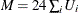. If M bytes are not available, PROC UNIVARIATE must process the data multiple times to compute all the statistics. This reduces the minimum memory requirement to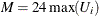.

Using the ROUND= option reduces the number of unique values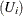, thereby reducing memory requirements. The ROBUSTSCALE option requires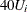bytes of temporary storage.

Several factors affect the CPU time:

• The time to create V tree structures to internally store the observations is proportional to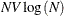.

• The time to compute moments and quantiles for the ith variable is proportional to.

• The time to compute the NORMAL option test statistics is proportional to N.

• The time to compute the ROBUSTSCALE option test statistics is proportional to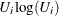.

• The time to compute the exact significance level of the sign rank statistic can increase when the number of nonzero values is less than or equal to 20.

Each of these factors has a different constant of proportionality. For additional information about optimizing CPU performance and memory usage, see the SAS documentation for your operating environment.